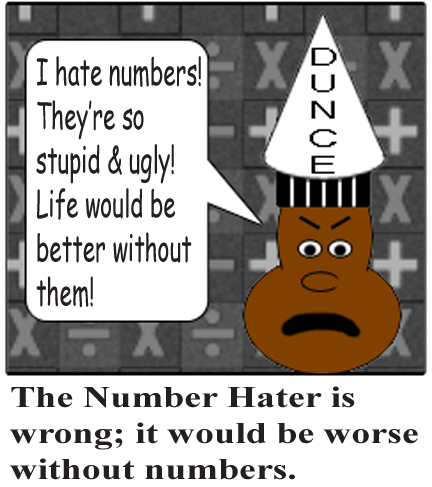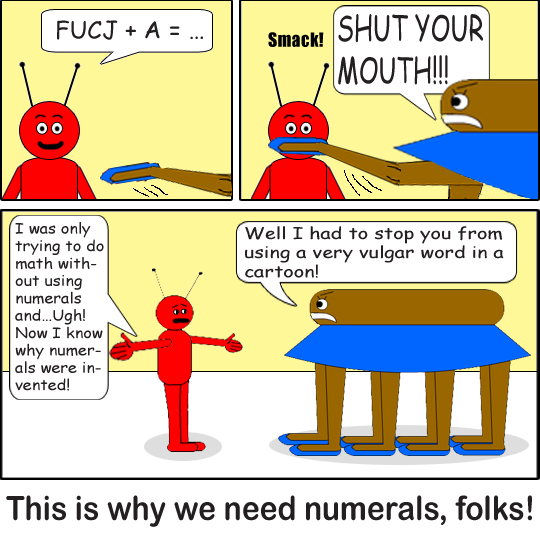Why Numbers Are Important

Imagine a world without numbers. Although there would be no math homework, there would also be several bad things in such a world. People would have a hard time counting how many things there are, and how big something is. There would be all sorts of important facts people wouldn't know if it weren't for numbers.A symbol that stands for a number is called a numeral. Without numerals, counting & measuring would be very problematic! Try using letters of the alphabet as numerals & you'll see how much more confusing math would be! (Well, they're already used as variables in Algebra & such sometimes, but numerals are different from variables!) Math is confusing enough for some of us already!

Suppose the letter A stands for the digit 1, B stands for the digit 2, C stands for the digit 3, etc., up to Z standing for 26. When you add A(1) to Z, then you get AA(27). Add A again to get AB(28). You can keep adding, but eventually you'll run into this horrible communication problem! This way, you are forced to use words(permutations of letters) as numbers; this includes cusswords! People would have a super-difficult time telling whether you're referring to the actual word or the "corresponding" number. Even if you spell the word, it would be hard to tell the difference. How miserable we would all be!However, 7 letters of the alphabet are used as Roman numerals: I for 1, V for 5, X for 10, L for 50, C for 100, D for 500, & M for 1,000. You know how to do Roman numerals already, don't you? A line is printed over V to stand for 5,000; the same is done with X to stand for 10,000; a line over L means 50,000; a line over C means 100,000; a line over D means 500,000; & a line over M means 1,000,000. Every line printed over a Roman numeral letter multiplies the numeral's number by 1,000; so 2 lines multiply the numeral by 1,000,000 or 1,000 squared. 3 lines multiply it by 1,000,000,000 or 1,000 cubed. It's multiplied by 1,000 to the power of the number of lines printed above it!

You can also spell words with Roman numerals,(like MIX, for example, which represents the number 1,009) but it's impossible to get cusswords this way so you don't have to worry about accidentally offending someone!(At least in the English language)What about the number zero(0) you ask? Well, back in ancient times, an underscore(_) was used to represent 0, so that answers your question easily! But still, all numbers are important to us & we need numerals to represent them to make our lives easier. Every number is equally important in mathematics, no matter how big or small it is; whether it's even or odd; or a fraction or an integer; real or imaginary, or whatever else!